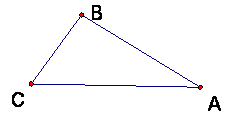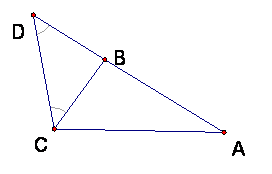## Triangle Inequality

Theorem:  In a triangle, the length of any side is less than the sum of the other two sides.

So in a triangle ABC, |AC| <  |AB| + |BC|.  (Also, |AB| < |AC| + |CB|; |BC| < |BA| + |AC|.)This is an important theorem, for it says in effect that the shortest path between two points is the straight line segment path. This is because going from A to C by way of B is longer than going directly to C along a line segment.

Proof:

We will add something to the figure that “straightens out” the broken path.  Construct point D on line AB so that BD = BC and so |AD| = |AB| + |BC|.Then since triangle BDC is isosceles by construction of D, then the base angles DCB and CDB are congruent.  But angle DCB is smaller than angle DCA; for this angle is contained inside angle DCA, since B is between D and A.

But this means that in the triangle ADC, Angle D is less than angle C, so for the opposite sides: |AC| <  |AD|. But |AD| = |AB| + |BC| by the ruler axiom.

QED

### “Triangle equality” and collinearity

Theorem: If A, B, C are distinct points in the plane, then |CA| = |AB| + |BC| if and only if the 3 points are collinear and B is between A and C (i.e., B is on segment AC).

Proof:

First we prove that the equality is true if B is between A and C.  Choose a ruler on the line AB; then the 3 points correspond to numbers a, b, c and either a <  b < c or c < b < a.

Suppose a  <  b < c.  Then |AC| = |c – a| = c – a = (c – b) + (b – a) = |c – b| + |b – a| = |AB| + |BC|.  This uses (carefully) the order of the numbers, so that |c – a| = c – a, because a < c, for example.  The case c > b > a gives the same result.

For the other direction, the converse, we must prove that if |AC| = |AB| + |BC|, then the points are collinear and B is between A and C.

First, the points must be collinear, for if they were not, then ABC would be a triangle and the triangle inequality would be true.  If the points are collinear, then as we saw from the ruler computation, B must be between A and C.  If this were not the case, and if, say A were between B and C, then |CB| would = |CA| + |AB|.  But we would still have |CA| = |AB| + |BC|, so together these would give |CB| = |AB| + |BC| + |AB|, or 0 = 2|AB|.  This is impossible for distinct points A and B.

QED

So in the end if A, B, C are distinct points on the plane, and |AC| is greater than or equal to each of |AB| and |BC|, then there are 2 possible cases and one impossible case.

|AC| < |AB| + |BC| (ABC is a triangle)

|AC| = |AB| + |BC|, (B is on segment AC)

|AC| > |AB| + |BC| (impossible)

Or in other words, for distinct points A, B, C, it is always true that |AC| is less than or equal to |AB| + |BC|, with equality occurring precisely when B is on segment AC.

Corollary: For any 4 non-collinear points A, B, C, D, the distance |AD| < |AB| + |BC| + |CD|.

Proof:

Either ABD is a triangle or ACD is a triangle (or both) because of non-collinearity.  Suppose ABD is a triangle.  Then |AD| < |AB| + |BD|.  Next get |BD| < or = |BC| + |CD| from BCD.

QED

Note:  This can be extended to any number of points using the same idea.